# Confidence interval credible interval and high density interval

• Slides: 11Confidence interval, credible interval and high density interval Xuhua Xia [email protected] ca http: //dambe. bio. uottawa. caMaximum likelihood illustration • The likelihood approach always needs a model. As a fish is either a male or a female, we use the model of binomial distribution, and the likelihood function is • The maximum likelihood method finds the value of p that maximizes the likelihood value. This maximization process is simplified by maximizing the natural logarithm of L instead: • The likelihood estimate of the variance of p is the negative reciprocal of the second derivative, Xuhua Xia Slide 2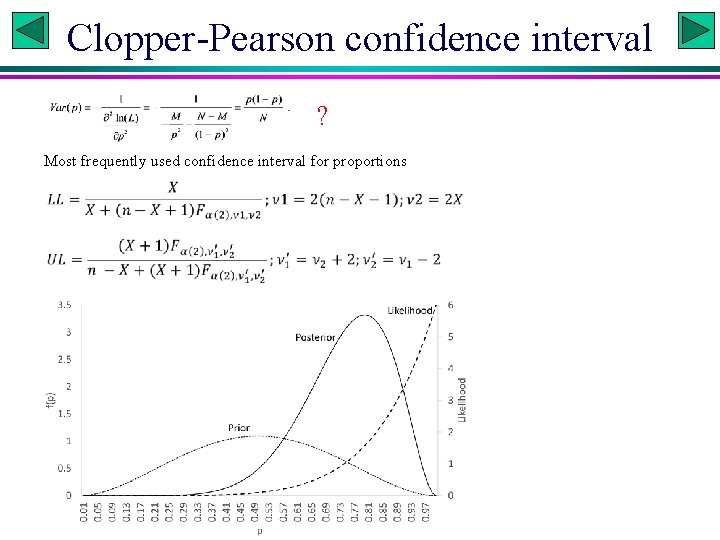Clopper-Pearson confidence interval ? Most frequently used confidence interval for proportions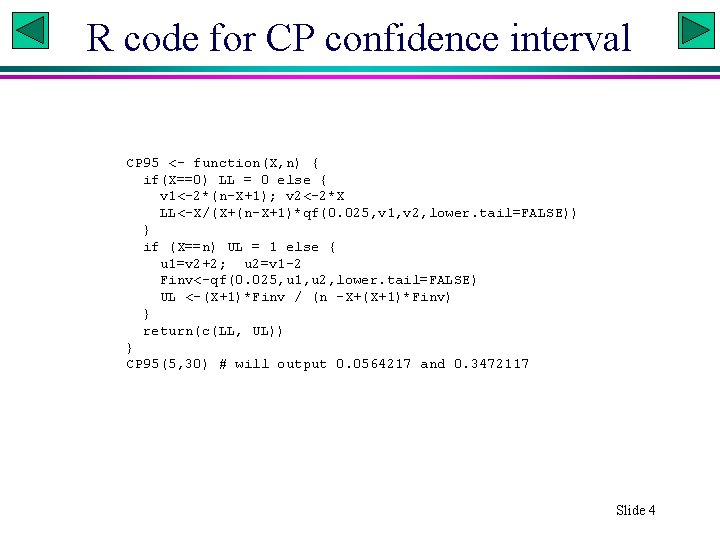R code for CP confidence interval CP 95 <- function(X, n) { if(X==0) LL = 0 else { v 1<-2*(n-X+1); v 2<-2*X LL<-X/(X+(n-X+1)*qf(0. 025, v 1, v 2, lower. tail=FALSE)) } if (X==n) UL = 1 else { u 1=v 2+2; u 2=v 1 -2 Finv<-qf(0. 025, u 1, u 2, lower. tail=FALSE) UL <-(X+1)*Finv / (n -X+(X+1)*Finv) } return(c(LL, UL)) } CP 95(5, 30) # will output 0. 0564217 and 0. 3472117 Slide 4Bayesian equivalents of C. I. • Posterior probability density function known – Credible interval (exact) – High density interval (exact) • Posterior probability density function unknown – Credible interval (approximate) – High density interval (approximate) Slide 5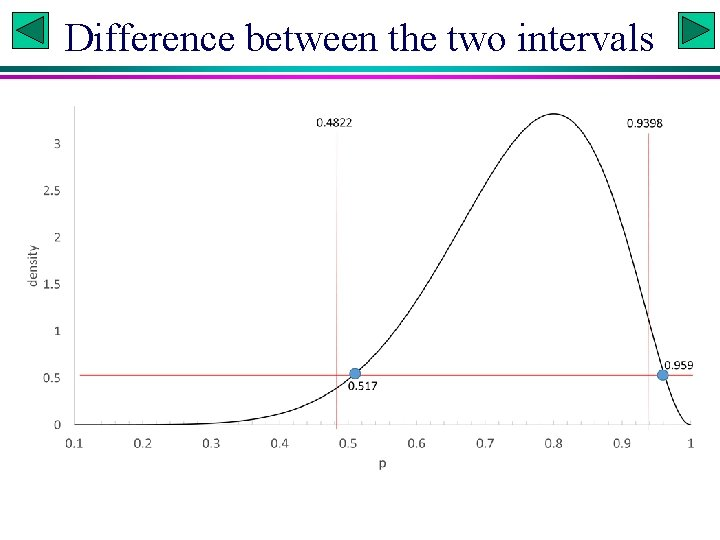Difference between the two intervals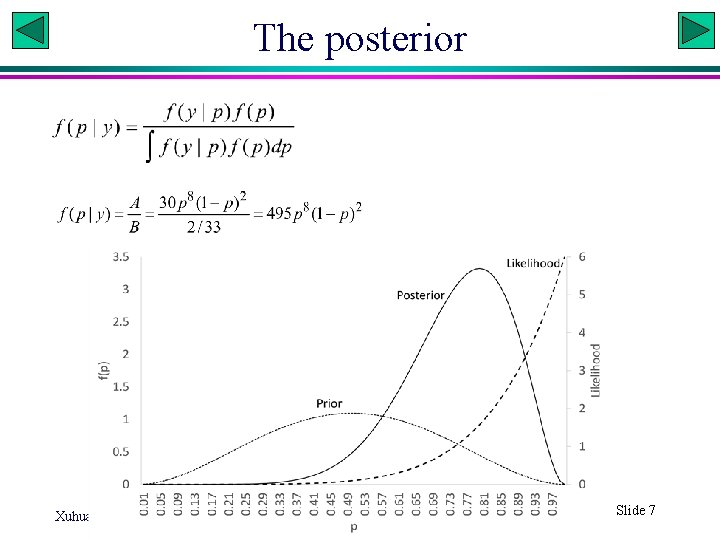The posterior Xuhua Xia Slide 7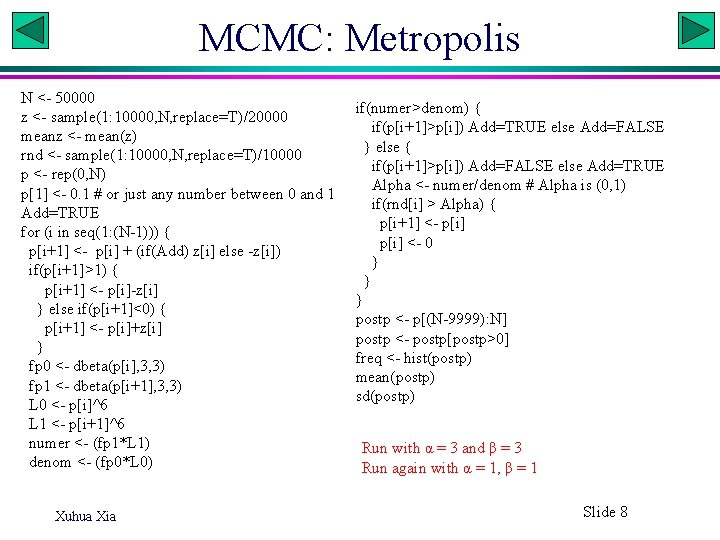MCMC: Metropolis N <- 50000 z <- sample(1: 10000, N, replace=T)/20000 meanz <- mean(z) rnd <- sample(1: 10000, N, replace=T)/10000 p <- rep(0, N) p <- 0. 1 # or just any number between 0 and 1 Add=TRUE for (i in seq(1: (N-1))) { p[i+1] <- p[i] + (if(Add) z[i] else -z[i]) if(p[i+1]>1) { p[i+1] <- p[i]-z[i] } else if(p[i+1]<0) { p[i+1] <- p[i]+z[i] } fp 0 <- dbeta(p[i], 3, 3) fp 1 <- dbeta(p[i+1], 3, 3) L 0 <- p[i]^6 L 1 <- p[i+1]^6 numer <- (fp 1*L 1) denom <- (fp 0*L 0) Xuhua Xia if(numer>denom) { if(p[i+1]>p[i]) Add=TRUE else Add=FALSE } else { if(p[i+1]>p[i]) Add=FALSE else Add=TRUE Alpha <- numer/denom # Alpha is (0, 1) if(rnd[i] > Alpha) { p[i+1] <- p[i] <- 0 } } } postp <- p[(N-9999): N] postp <- postp[postp>0] freq <- hist(postp) mean(postp) sd(postp) Run with α = 3 and β = 3 Run again with α = 1, β = 1 Slide 8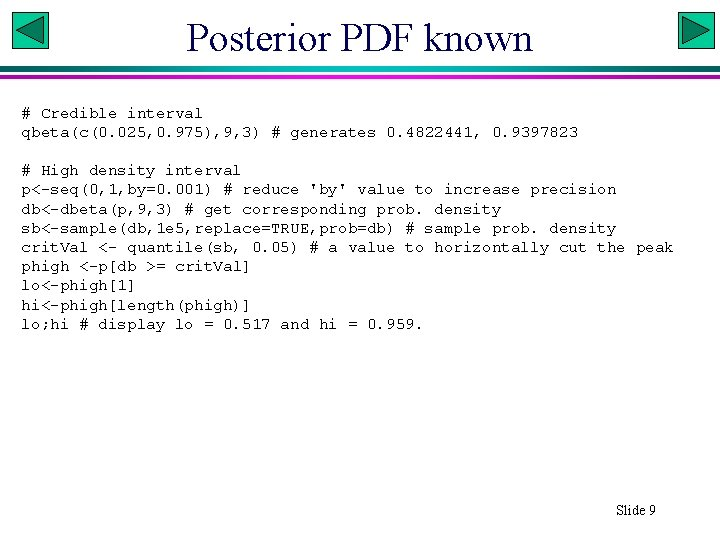Posterior PDF known # Credible interval qbeta(c(0. 025, 0. 975), 9, 3) # generates 0. 4822441, 0. 9397823 # High density interval p<-seq(0, 1, by=0. 001) # reduce 'by' value to increase precision db<-dbeta(p, 9, 3) # get corresponding prob. density sb<-sample(db, 1 e 5, replace=TRUE, prob=db) # sample prob. density crit. Val <- quantile(sb, 0. 05) # a value to horizontally cut the peak phigh <-p[db >= crit. Val] lo<-phigh hi<-phigh[length(phigh)] lo; hi # display lo = 0. 517 and hi = 0. 959. Slide 9Approximate posterior PDFPosterior PDF unknown # Quick and dirty credible interval quantile(postp, c(0. 025, 0. 975)) # generates credible interval # More refined method res<-hist(postp, 100) x<-res\$mids; dx<-res\$density fit 25<-loess(dx~x, span=0. 25) # higher span leads to more smooth fit p<-seq(min(x), max(x), by=0. 001) # reduce 'by' value to increase precision dp<-pmax(0, predict(fit 25, data. frame(x=p))) # pmax changes all negative values to 0. samp<-sample(p, 1 e 5, replace=TRUE, prob=dp) quantile(samp, c(0. 025, 0. 975)) # produce credible interval samp <- sample(dp, 1 e 5, replace = TRUE, prob = dp) # sample probability densities crit <- quantile(samp, 0. 05) # a horizontal line to cut the peak phigh <-p[dp >= crit] lo<-phigh; hi<-phigh[length(phigh)] lo; hi # produce high density interval Slide 11